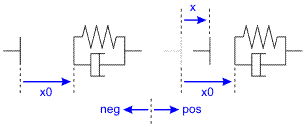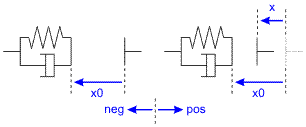﻿ 20-sim webhelp > Library > Iconic Diagrams > Mechanical > Translation > Components > Collision-Relative

Collision-Relative

Library

Iconic Diagrams\Mechanical\Translation\Components

Right

Left

Use

Domains: Continuous. Size: 1-D. Kind: Iconic Diagrams (Translation).

Description - Right

This model represents an collision of an object with another object. It can be used to indicate a possible collision to the right of an object.The collision force is modeled by a spring and damper:

p.F = if x > x0 then k*(x-x0) + d*limit(p.v,0,1e20) else 0 end;

with a stiffness k and damping d. The limit function is used to prevent the damper force to become negative. The initial position of the spring is indicated by x0 (see the figure above). Note that the positive direction is to the right. If x is larger that x0, the two sides have collided and are in contact. The collision model has separate high and low ports. The equations are

p.F = p_high.F = p_low.F

p.v = p_high.v - p_low.v

Interface - Right

Ports

Description

p_high

p_low

Two ports of the collision model.

fixed force out

Variables

x

extension of the spring [m]

Parameters

x0

k

d

unstretched spring position

stiffness [N/m]

damping [N.s/m]

Initial Values

x_initial

The initial extension of the spring [m].

Description - Left

This model represents an collision of an object with another object. It can be used to indicate a possible collision to the left of an object.The collision force is modeled by a spring and damper. Note that the positive direction is to the right, so the initial position of the spring x0 has a negative value in the figure above. If x is smaller that x0, the two sides have collided and are in contact.

Interface - Left

Ports

Description

p_high

p_low

Two ports of the collision model.

fixed force out

Variables

x

extension of the spring [m]

Parameters

x0

k

d

unstretched spring position

stiffness [N/m]

damping [N.s/m]

Initial Values

x_initial

The initial extension of the spring [m].